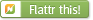Forum: DSP What is the effect of reducing nfft less than the signal length

 Author: Usman A. (Company: LUMS) (usmanashraf678) Posted on: 2018-02-12 09:10

Rate this post
 0 ▲ useful ▼ not useful
Dear members,
I am analyzing a signal in matlab by taking its fft. The singal has 200
samples and I am currently taking nfft = 256. I understand that this
would result in zero padding. Now, I want to try to reduce the nfft to
128, 64 etc. I wanted to ask what should I expect to happen with the
fft.

Matlab says
for Y = fft(X,n)
If X is a vector and the length of X is greater than n, then X is
truncated to length n. What would this actually result in? Can anyone

 Author: Andreas S. (andreas) (Admin)Posted on: 2018-02-13 20:11

Rate this post
 0 ▲ useful ▼ not useful
You will get a reduced frequency resolution, and, depending on your
input vector, windowing artifacts (you can consider truncation as
equivalent to applying a rectangular window).

 Author: Martin B. (Guest) Posted on: 2018-02-25 17:46

Rate this post
 0 ▲ useful ▼ not useful
You may also use Nfft = 200

I think in matlab you can use any number Nfft which is a factor of
2,3,5.
It is not neccesary to use Nfft = 2^n, You may also use
Nfft=200=2*2*2*5*5

Most fft librraries are able to use these numbers of Nfft (fftw, kissFFT
..)

• $formula (LaTeX syntax)$